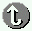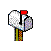## Calculating Availability - Examples

(IBM PC Server Technology and Selection Reference SG24-4760-02 Aug-1997 U.S.)

Calculating Availability - Examples

When establishing a goal for availibility, trying to get realistic figures is very important. Most Manufacturers (hardware and software) will not publish available figures, since they are dependent on the environment in which the machine operates. One figure you might find published, in the Mean Time Between Failure  or MBTF value. The MBTF is defined as being the amount of time a piece of hardware can run, without breaking down. Since these MBTF are based on statistical models, the are theoretical. The theoretical MTBF is actually the average time a component would run without failing, tested on an enormous population, and without including some common breakdown reasons. It should be clear that the theoretical MTBF does not reflect the operational MTBF.

So, what can we do with this MTBF ? A first approximate figure we can calculate is the probability of survival . This figure gives the chance a component will still function after a certain amount of time, based on the MTBF. The formular used is:

R = e * (Useful Life / MTBF) = Probability of Survival

With Useful Life equal to the number of years the hardware is supposed to work.
Let's have an example:

The IBM Ultrastar ES 2.16 GB Ultra SCSI Hard Drive has a projected MTBF of 800.00 power-on hours. A first step to take is converting these power-on hours to actual years.
There are two possibilities:

•  1 year equals 8760 hours, typical for large PC Servers (100% duty cycle).
•  1 year equals 6240 hours, typical for small networks (71% duty cycle).

We'll take a 100% duty cycle, so 800.000 hours converts approximately into 91 years. We want to know the probability of survival after 3 years. This gives us the following result:

R = EXP(-3/91) = 97%

A second number that might interest us is the Annual Failure Rate . The AFR reflects the number of components that will fail each year. It can be calculated using the following two formulas:

AFR = (power-on hours / MBTF) * 100%

So, lets say you have 1000 hard disks installed. Using the above figures, the AFR totals to:

AFR = (8760 / 800.000) * 100% = 1.1 %

This means that of the 1000 installed units, approximately 11 will fail annually.

There are some important remarks to make here:
•  Early life failure can increase the number of failures during the first year.
•  The statistical approaches are based upon a very large population. If the number of installed units is small, large  fluctuations can occur.
•  When calculating the MTBF of a collection of components that are combined in such a way that failure of one of them will  bring the others down

(for example, a RAID-0 configuration), the following formula should be used:

Total MTBF = (MTBF_1 * MTBF_2 * .... * MTBF_n) / (MTBF_1 + MTBF_2 + .... + MTBF_n)

This total MTBF will be smaller than the individual MTBFs.

Back toFeel free - send afor any BUG on this page found - Thank you.